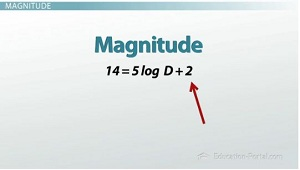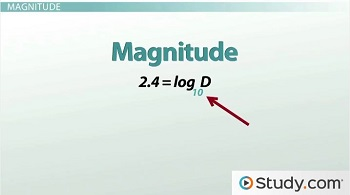# How to Solve Logarithmic Equations

An error occurred trying to load this video.

Try refreshing the page, or contact customer support.

Coming up next: What Is a Factorial?

### You're on a roll. Keep up the good work!

Replay
Your next lesson will play in 10 seconds
• 0:06 Magnitude
• 3:18 Product Property
• 4:55 Exponentiating
• 6:08 Lesson Summary

Want to watch this again later?

Timeline
Autoplay
Autoplay
Speed

#### Recommended Lessons and Courses for You

Lesson Transcript
Instructor: Luke Winspur

Luke has taught high school algebra and geometry, college calculus, and has a master's degree in education.

If you're ever picking out a telescope to see your favorite planet, make sure you do the math first! Learn how to solve the logarithmic equation that will get you the size telescope you want.

## Magnitude

I'm not going to lie; I'm kind of a nerd, and I got really into science fiction when I was just a little kid. Maybe it helps that I was named after Luke Skywalker, but, either way, starting in elementary school, I couldn't stop thinking about the Force, spaceships and our solar system. My favorite planet was Pluto (although, I suppose it's more of an ex-planet now), but either way I wanted to get a telescope so I could see it. My parents and I found a really cheap one at a store close to my house, but much to my dismay, when I got home and tried it out it wasn't nearly good enough.As it turns out, the telescope I got could only see stars with an apparent magnitude of 8, but what I needed was one that could see stars with an apparent magnitude of 14. Too bad I wasn't a math teacher yet and didn't know the equation magnitude equals 5 times the log of diameter plus 2 (or M = 5 log D + 2). This is the equation that tells you what magnitude star you can see with a lens that has a diameter of D millimeters. If I had been a math teacher, I would have known that I could substitute 14 in for M and then solve the equation for D. The resulting answer would tell you how big your lens would need to be in order to see stars with an apparent magnitude of 14.

So, how big of a telescope did I actually need? Well, it's again our job to use inverse operations to get the D by itself in this equation. We need to start peeling the layers off of this equation one at a time in reverse order, starting with the outermost step in this equation, which is the + 2. I can undo addition with subtraction, and subtracting 2 from both sides gives me 12 = 5 log D. Now, the outermost layer is 5 times. I undo multiplication with division, dividing both sides by 5, and I get 2.4 = log D. Now, I need to undo a log, and this is where the new skill comes into play. We do know that the inverse operation to a log is an exponential, so I need to use an exponential to undo this log. I need to pick my exponential to be the same type of log that this is - meaning I need to be taking the same base log and exponential in order to undo them. This log is blank, which means there's an imaginary base 10 in there, so I need to do the exponential base 10 on both sides.This is a little strange; we haven't actually done this before. A common mistake I see is people try to raise the log to the 10th power, but that's not exactly what we want. An exponential has the variable in the exponent, so the 10 shouldn't be the exponent; the log D should be the exponent. What I do is write 10 to the bottom left of the log, and that is my base, so I get 10(log D). Whatever I do to one side, I have to do to the other side, so I have 10(2.4) = 10(log D). The 10(log10) undoes, because the exponential and log are inverse operations, so I just get 10(2.4) = D. If I plug 10(2.4) into my calculator, I get ~251. That means that I needed a telescope that had a lens with a diameter of 251 millimeters. That's almost 10 inches, which is a pretty big telescope! Maybe it's for the best that I didn't know this equation, because if I would've asked my parents for something that big I would've been rejected for sure.

## Product Property

We can use similar skills to solve even harder logarithmic equations, like log2 (x) + log2 (5) = 10. This is more difficult than our telescope example because there are two logs instead of one. This means we'll get a chance to practice our property skills as well as our solving skills all in one problem! Before I can solve this equation like we did before, I need to use the product property to combine the two logs into one. I picked the product property because I saw two logs separated by addition. When I see that, the product property tells me that I can condense them into one log where the two things on the inside are now multiplied. So, instead of log2 (x) + log2 (5), I combine them into one, multiply 5 and x and I get log2 (5x) = 10.

To unlock this lesson you must be a Study.com Member.

### Register to view this lesson

Are you a student or a teacher?

### Unlock Your Education

#### See for yourself why 30 million people use Study.com

##### Become a Study.com member and start learning now.
Back
What teachers are saying about Study.com

### Earning College Credit

Did you know… We have over 160 college courses that prepare you to earn credit by exam that is accepted by over 1,500 colleges and universities. You can test out of the first two years of college and save thousands off your degree. Anyone can earn credit-by-exam regardless of age or education level.